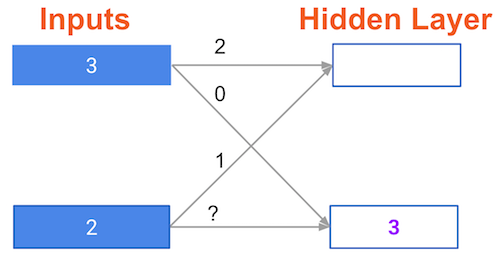# A round of backpropagation

In the network shown below, we have done forward propagation, and node values calculated as part of forward propagation are shown in white. The weights are shown in black. Layers after the question mark show the slopes calculated as part of back-prop, rather than the forward-prop values. Those slope values are shown in purple.

This network again uses the ReLU activation function, so the slope of the activation function is 1 for any node receiving a positive value as input. Assume the node being examined had a positive value (so the activation function's slope is 1).What is the slope needed to update the weight with the question mark?